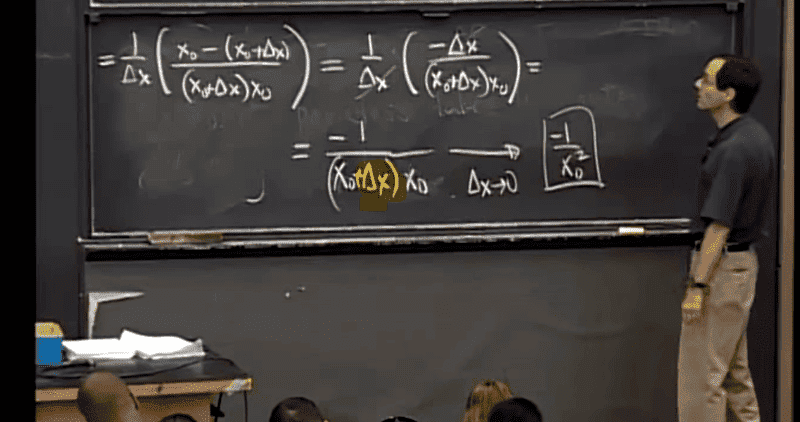# What am I missing here in this difference quotient problem?

• I
• JR Sauerland

#### JR Sauerland

Gold Member
I've highlighted the part in yellow I don't understand. He apparently 'drops' Δx in the last line, but doesn't display how. What I do know is that he is taking the limit as Δx→0, or as Delta x approaches zero. I'm simpy missing what he did though to drop the Δx. I mean, I understand he distributed x into the parentheses to get x0 squared, but not how he just dropped the Δx.

Any insight?The work on the chalkboard looks good. The first pair of delta x which the lecturer canceled acted as a factor of 1. The highlighted delta x would become increasingly, vanishingly small and so is taken as near enough to zero. The lecturer has shown the limit of the original expression as delta x approaches zero.

(minor edit)

Last edited:
He did not drop anything. He DID do a cross-cancellation of a delta X on top and a delta X on bottom. He even shows lines through them to emphasize this.

If you are asking about the very last bit of "= -1 / (X0) ^ 2 then that is, as he clearly states, a limit as delta X approaches zero.

The highlighted delta x sub zero would become increasingly, vanishingly small and so is taken as near enough to zero. The lecturer has shown the limit of the original expression as delta x sub zero approaches zero.

Does that mean that since delta x is approaching zero, it is just left out because it is so small? I guess I just don't understand at all.

Does that mean that since delta x is approaching zero, it is just left out because it is so small? I guess I just don't understand at all.
Yes, that is exactly what it means. The concept of the limit is that you see what would happen if it actually GOT to the limiting amount.

Does that mean that since delta x is approaching zero, it is just left out because it is so small? I guess I just don't understand at all.
Yes, that is exactly what it means. The concept of the limit is that you see what would happen if it actually GOT to the limiting amount.
Understand that in the shown example, letting delta x become zero does not make the denominator part of the expression undefined.

(Reminder: I made a minor edit to my response.)

Um, do you have a textbook or anything? It doesn't seem that you grasp limits at all or what they're supposed to be. If you don't understand things like this, it's silly to move on. You need to go back in your book and read it from the start again until you grasp what a limit is.